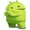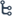பாடசாலை வலைதளத்தின் New Android App ஐ Download செய்து பயன்படுத்தவும்! - https://play.google.com/store/apps/details?id=com.padasalai.padasalai

••## CBSE Class 8 Maths - New Syllabus 2020 - 2021 DownloadCBSE Class 8 Maths Syllabus – 2020-2021

 Chapter Topics Contents 1 Rational Numbers 1.1 Introduction 1.2 Properties of Rational Numbers 1.3 Representation of Rational Numbers on the Number Line 1.4 Rational Number between Two Rational Numbers 2 Linear Equations in One Variable 2.1 Introduction 2.2 Solving Equations which have Linear Expressions on one Side and Numbers on the Other Side 2.3 Some Applications 2.4 Solving Equations having the Variable on both sides 2.5 Some More Applications 2.6 Reducing Equations to Simpler Form 2.7 Equations Reducible to the Linear Form 3 Understanding Quadrilaterals 3.1 Introduction 3.2 Polygons 3.3 Some of the Measures of the Exterior Angles of a Polygon 3.4 Kinds of Quadrilaterals 3.5 Some Special Parallelograms 4 Practical Geometry 4.1 Introduction 4.2 Constructing a Quadrilateral 4.3 Some Special Cases 5 Data Handling 5.1 Looking for Information 5.2 Organising Data 5.3 Grouping Data 5.4 Circle Graph or Pie Chart 5.5 Chance and Probability 6 Squares and Square Roots 6.1 Introduction 6.2 Properties of Square Numbers 6.3 Some More Interesting Patterns 6.4 Finding the Square of a Number 6.5 Square Roots 6.6 Square Roots of Decimals 6.7 Estimating Square Root 7 Cubes and Cube Roots 7.1 Introduction 7.2 Cubes 7.3 Cubes Roots

CBSE Class 8 Maths Syllabus – 2020-2021

 Chapter Topics Contents 8 Comparing Quantities 8.1 Recalling Ratios and Percentages 8.2 Finding the Increase and Decrease Percent 8.3 Finding Discounts 8.4 Prices Related to Buying and Selling (Profit and Loss) 8.5 Sales Tax/Value Added Tax/Goods and Services Tax 8.6 Compound Interest 8.7 Deducing a Formula for Compound Interest 8.8 Rate Compounded Annually or Half Yearly (Semi-Annually) 8.9 Applications of Compound Interest Formula 9 Algebraic Expressions and Identities 9.1 What are Expressions? 9.2 Terms, Factors and Coefficients 9.3 Monomials, Binomials and Polynomials 9.4 Like and Unlike Terms 9.5 Addition and Subtraction of Algebraic Expressions 9.6 Multiplication of Algebraic Expressions: Introduction 9.7 Multiplying a Monomial by a Monomial 9.8 Multiplying a Monomial by a Polynomial 9.9 Multiplying a Polynomial by a Polynomial 9.10 What is an Identity? 9.11 Standard Identities 9.12 Applying Identities 10 Visualizing Solid Shapes 10.1 Introduction 10.2 View of 3D-Shapes 10.3 Mapping Space Around Us 10.4 Faces, Edges and Vertices 11 Mensuration 11.1 Introduction 11.2 Let us Recall 11.3 Area of Trapezium 11.4 Area of General Quadrilateral 11.5 Area of Polygons 11.6 Solid Shapes 11.7 Surface Area of Cube, Cuboid and Cylinder 11.8 Volume of Cube, Cuboid and Cylinder 11.9 Volume and Capacity 12 Exponents and Powers 12.1 Introduction 12.2 Powers with Negative Exponents 12.3 Laws of Exponents 12.4 Use of Exponents to Express Small Numbers in Standard Form

CBSE Class 8 Maths Syllabus – 2020-2021

 Chapter Topics Contents 13 Direct and Inverse Proportions 13.1 Introduction 13.2 Direct Proportion 13.3 Inverse Proportion 14 Factorisation 14.1 Introduction 14.2 What is Factorisation? 14.3 Division of Algebraic Expressions 14.4 Division of Algebraic Expressions Continued (Polynomial / Polynomial) 14.5 Can you Find the Error? 15 Introduction to Graphs 15.1 Introduction 15.2 Linear Graphs 15.3 Some Applications 16 Playing with Numbers 16.1 Introduction 16.2 Numbers in General Form 16.3 Game with Numbers 16.4 Letters for Digits 16.5 Test of Divisibility Exam